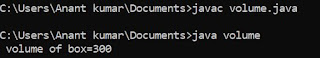## Wednesday, 13 June 2018

### Program to print first n terms of fibonacci series by user define function in C

# include <stdio.h>
void  fibo (int ,int ,int) ;
void main ()
{
int a=0,b=1,n ;
clrscr () ;
printf ("\n How many terms of fibo series you want to print:") ;
scanf ("%d", & n) ;
fibo (a,b,n) ;
getch () ;
}
void fibo (int a,int b,int n)
{
int counter =0 ;
int sum ;
printf ("\n %d", a) ;
printf ("\n %d", b) ;
while (counter < n-2)
{
sum =a+b ;
printf ("\n sum =%d", sum) ;
a=b ;
b=sum ;
counter++ ;
}
}

DRY RUN :
If n = 3 , a = o , b = 1 , counter = 0
( 0 < 3 ) TRUE
Sum = a + b ;  0 + 1 = 1
( 1 < 3 ) TRUE                        Counter = 1
Sum = a + b ;  1 + 1 = 2
( 2 < 3 ) TRUE                         counter = 2
Sum = a + b ;  1 + 2 = 3
( 3 < 3 )  FALSE                       counter = 3

The output of this program is comes as 0 , 1 , 1 , 2 ,3 .